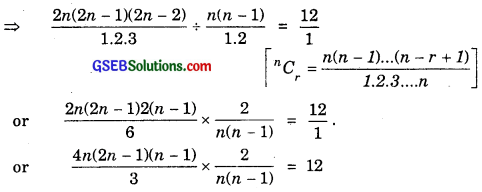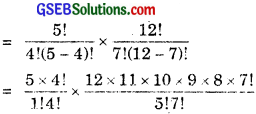# GSEB Solutions Class 11 Maths Chapter 7 Permutations and Combinations Ex 7.4

Gujarat Board GSEB Textbook Solutions Class 11 Maths Chapter 7 Permutations and Combinations Ex 7.4 Textbook Questions and Answers.

## Gujarat Board Textbook Solutions Class 11 Maths Chapter 7 Permutations and Combinations Ex 7.4

Question 1.
If nC8 = nC2. Find nC2.
Solution:
We have: nCr = nCn-r
nC2 = nCn-2
nC8 = nCn-2 ⇒ n – 2 = 8 or n = 10.
nC2 = 10C2 = $$\frac{10×9}{1×2}$$ = 45.Question 2.
Determine n, if
(i) 2nC3 : nC2 = 12 : 1
(ii) 2nC3 : nC3 = 11 : 1
Solution:
2nC3 : nC2 = 12 : 12n – 1 = 9, 2n = 10 or n = 5.

(ii) 2nC3 : nC3 = 11 : 1∴ 4(2n – 1) = 11(n – 2)
or 8n – 4 = 11n – 22
or 3n = 18 ⇒ n = 6.Question 3.
How many chords can be drawn through 21 points on a circle?
Solution:
We get a chord by joining two points. If p is the number of chords from 21 points, then
p = C(21, 2) = $$\frac{21!}{2!(21 – 2)!}$$ = $$\frac{21!}{2!19!}$$ = $$\frac{21×20(19)!}{2×1(19!)}$$
= 21 × 10 = 210 chords.

Question 4.
In how many ways can a team of 3 boys and 3 girls be selected from 5 boys and 4 girls?
Solution:
3 boys out of 5 boys can be selected in 5C3 ways.
3 girls out of 4 girls can be selected in 4C3 ways.
∴ Number of ways in which 3 boys and 3 girls can be selected
= 5C3 × 4C3 = 5C2 × 4C1.
= $$\frac{5×4}{1×2}$$ × $$\frac{4}{1}$$ = 40. [∵ nC2 = nCn-2.Question 5.
Find the number of ways of selecting 9 balls from 6 red balls, 5 white balls and 5 blue balls, if each selection consists of 3 balls of each colour?
Solution:
The number of ways of selecting 3 red balls out of 6 red balls = 6C3.
The number of ways of selecting 3 white balls out of 6 white balls = 5C3.
The number of ways of selecting 3 blue balls out of 5 blue balls = 5C3.
The number of ways of selecting 3 balls of each colour
= 6C3 × 5C3 × 5C3 = 5C3 × 5C2 × 5C2.
= $$\frac{6×5×4}{1×2×3}$$ × $$\frac{5.4}{1×2}$$ × $$\frac{5.4}{1.2}$$.
= 20 × 10 × 10 = 2000.

Question 6.
Determine the number of 5 cards combinations out of a deck of 52 cards, if there is exactly one ace in each combination?
Solution:
One ace will be selected from four aces and four cards will be selected from (52 – 4 =) 48 cards. If p is the required number of ways, then
p = C(4, 1) × C(48, 4)= 4 × 2 × 47 × 46 × 45 = 778320 ways.Question 7.
In how many ways, can we select a cricket eleven from 17 players in which only 5 players can bowl, if each cricket eleven must include exactly 4 bowlers?
Solution:
Four bowlers can be selected from the five bowlers and seven players can be selected from 12 players (17 – 5 = 12). If ‘p’ is the number of ways of selecting the cricket eleven, then
p = C(5, 4) × C(12, 7)= 3960.

Question 8.
A bag contains 5 black and 6 red balls. Determine the number of ways in which 2 black and 3 red balls cars be selected?
Solution:
The number of ways in which 2 black balls out of 5 black balls can be selected = 5C2.
The number of ways in which 3 red balls out of 6 red balls can be selected = 6C3.
The number of ways in which 2 black and 3 red balls can be selected
= 5C2 × 6C3 = $$\frac{5×4}{1×2}$$ × $$\frac{6×5×4}{1×2×3}$$.
= 10 × 20 = 200.Question 9.
In how many ways, can a student choose a programme of 5 courses, if 9 courses are available and 2 courses are compulsory for every student?
Solution:
Out of available nine courses, two are compulsory. Hence, the student is free to select 3 courses out of 7 remaining courses. If p is the number of ways of selecting 3 courses out of 7 courses, then
p = C(7, 3)
= $$\frac{7!}{3!(7 – 3)!}$$ = $$\frac{7!}{3!4!}$$ = $$\frac{7×6×5×4!}{3×2×1×4!}$$
= 7 × 5 = 35 ways.